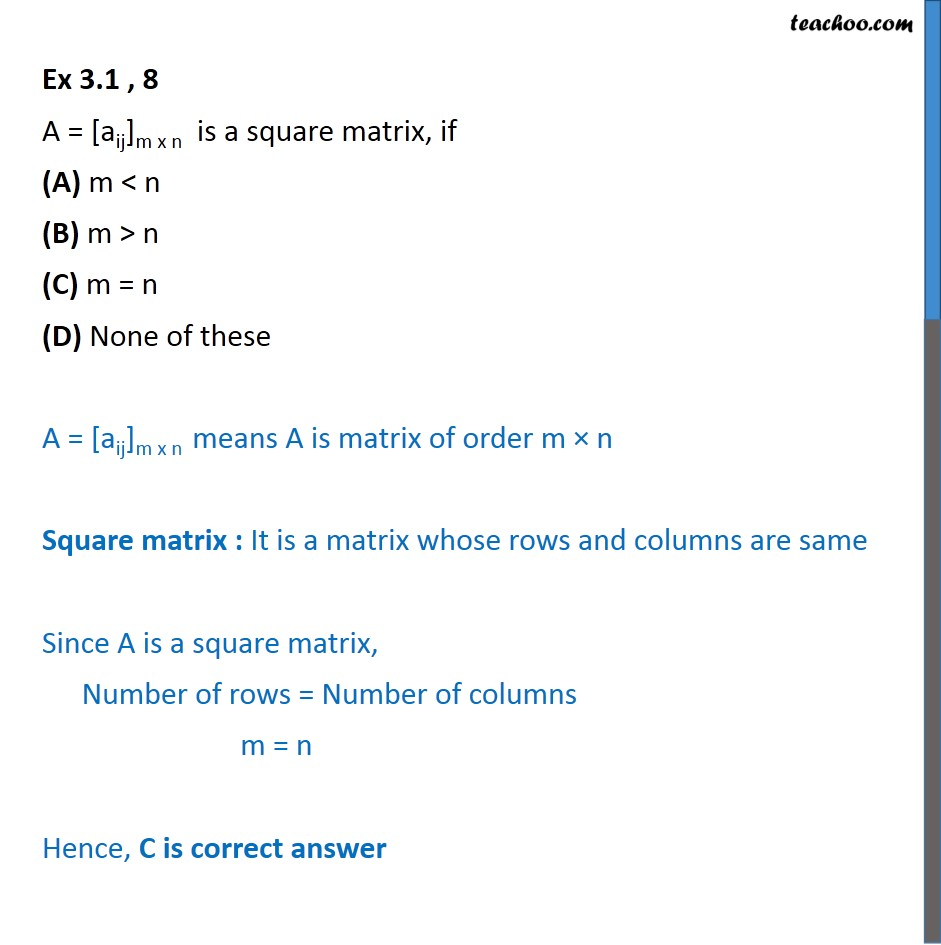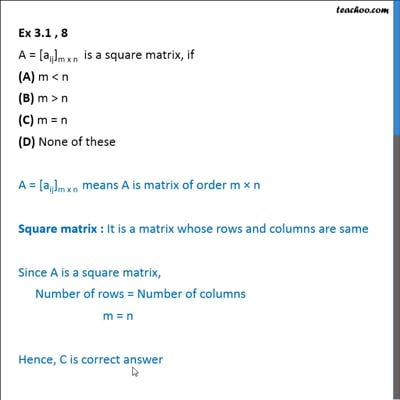Ex 3.1

Chapter 3 Class 12 Matrices
Serial order wiseThis video is only available for Teachoo black users

Solve all your doubts with Teachoo Black (new monthly pack available now!)

### Transcript

Ex 3.1 , 8 A = [aij]m x n is a square matrix, if (A) m < n (B) m > n (C) m = n (D) None of these A = [aij]m x n means A is matrix of order m × n Square matrix : It is a matrix whose rows and columns are same Since A is a square matrix, Number of rows = Number of columns m = n Hence, C is correct answer